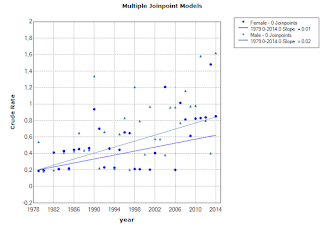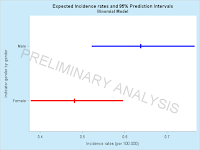top of page
Search

# Modeling gender and age adjusted incidence rates

National Health Institute (NHI) provides a tool box for calculation of cancer incidence and percentage change. Their algorithm for Jointpoint Trend Analysis is well-documented but does not provide the best tool at hand for most problems. The normal approximation is not the most optimal choice for situations with a low incidence rate in which I would recommend to apply modern logistic regression algorithms which are far more versatile. In the logistic regression model, either direct or indirect incidence rates are modeled using population numbers by age, year, and gender and number of cases specific to the given year, gender, and age group. There are no missing data if the registry is complete, compared to the normal model in which we cannot use data points for years/age-gender groups with no events and perform simulation to get approximate estimates.The difference between careful parametrization in a binomial regression model and the plug-and-play functionality of the NHI suite becomes obvious in an example in which we look at cancers in children. Data source: NORDCAN

Logistic regression models. Joint point model (left) using stepwise linear gender specific regression models and polynomial models (right) using gender specific polynomial regression models.Graphs with gender specific 95% prediction limitsR-script Data Extraction

SAS program

Joint Point Model based on software from NIH The estimation procedure does not allow zero-counts, which introduces bias.Furthermore, errors are approximate normal distributed.The logistic regression model predicts a total of 190 cancer cases during the period 1979-2014, whereas the Jointpoint trend program from NHI predicts 158 cases of cancer when adjusting for calendar year. Binomial model estimates a total combined incidence rate of 0.57 (per 100,000) corrected for calendar year, whereas the Jointpoint trend analysis program yields an incidence rate of 0.47 (per 100,000). We observe a total of 33,679,014 person years. We have used actual connective tissue cancer incidence counts for Danes age 0 to 24 from the NORDCAN register of gender specific incidence rates with a total of 189 cases in the period 1979-2014.